# How to do Differentiation with our Computers?

0
2130“Hidden in the plain sight.”

Dictionary says that the meaning of the above phrase as “being unnoticeable, by staying visible in a setting that masks presence”. Do you know that you cannot take a picture of the Eiffel tower at night? There are a lot of things in our life like this, that has slipped our conscious self and is still lying around waiting to surprise us.

What is 2 + 3?

Yeah, it’s 5. Now write a pseudo code for the sum of two number.

```a = 2
b = 3
c = a + b```

What is 2x + 3, when x = 6 ?
That’s correct, it is 15. Now write a pseudo code for the product of two numbers.

```x = 6
y = 2*x + 3```

Doing good till now? Now, what is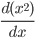?
If your high school math works properly, the answer must be. Now, like earlier, write a pseudo code that can find the derivative of the function.

```y = something
if(answer == "I dont know"):
print(“BOOM! Suddenly, it is not as easy as you thought.”)```

All our life, we have not paid enough attention to this situation and just imagined that it must exist somehow. This situation has been hiding in our plain site since we started learning calculus. But turns out it’s an area of research by in itself.

In this article, I have tried to address the existing solutions to this issue with their pros and cons.

There are three ways to go about this problem :

• Numerical differentiation
• Symbolic differentiation
• Automatic differentiation

Numerical Differentiation:

It is nothing but the differentiation method through limits that they taught us in high school. The equation goes something like this.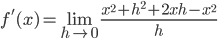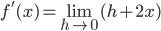But there is a problem with this method,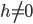but. Which means, h can be either 0.000001 or 0.000000000001. So, the final answer is approximated. If this is done once, we can use error correction and solve it but in Neural Networks, this is done millions of time, which adds of the error and ultimately gives out an incorrect solution.

The pseudo code for the Numerical differentiation is as follows:

```h = 0.0000001
x = 5
print((((x+h)^2)+x^2)/h)```

If you notice the code properly, it is clear that we should find the derivative of the function manually, convert it to expression and code it. So, for this method to work properly, we should know what the function is and hard code it, as a result, we cannot just randomly input a function and expect it to return the answer.

Popular Library: numpy

##### Since the “answer is approximated”, this cannot be used as an efficient way to find derivative in Neural Networks.

Symbolic Differentiation:
This is the type of differentiation we have all been using in our daily lives. Remember the derivatives of a few basic functions and apply the rules like,

and solve using Pen and paper.

This method provides a perfectly correct result but it suffers from a problem known as Expression Swell when the function is a bit large.
So, What is this so-called expression swell?

We have noticed this expression swell when we were introduced to Calculus but thought that it was meant to be that way and got used to it. By using the above rules finding the derivatives of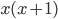,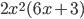,might seem fairly simple but you will start noticing the issue when you try finding the derivative of.

It’s derivative requires us to apply the above rules multiple times which results in the following expression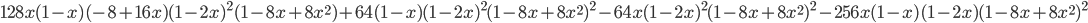which is a big swollen expression and compliments the meaning of the term Expression Swell.
This has never been a problem for us humans but computers have a hard time keeping track of it and therefore decreases it’s efficiency drastically.

The pseudo code for the Symbolic Differentiation is as follows :

```x = 2
print(128*x*(1-x)*(-8+16*x)*pow((1-2*x),2)*(1-8*x+8*pow(x,2))+64*(1-x)*pow((1-2*x),2)*pow((1-8*x+8*pow(x,2)),2)-64*x*pow((1-2*x),2)*pow((1-8*x+8*pow(x,2)),2)-256*x*(1-x)*(1-2*x)*pow((1-8*x+8*pow(x,2)),2))```

If you notice the code properly, like in numerical differentiation we should find the derivative of the function manually, convert it to expression and code it. Even though the answer is correct, it would not be efficient to do so with such a big expression.

Popular Library: sympy

##### Symbolic differentiation might result in “Expression Swell”. As a result, it cannot be used to find a derivative in Neural Networks.

When all the common ways that we know have defects in them and cannot be used in finding derivatives efficiently, then what is the solution for this?

Automatic Differentiation:
One of the most efficient ways to differentiate using a computer is called “Automatic Differentiation”. There a kind of a mystery cloud around it, which makes it hard to understand but when you understand it properly, it is just the chain rule that we used in our high school.
Revisiting Chain Rule :
Let’s suppose, we have a function,, the derivative of it is. To arrive at that answer, we would use the following chain rule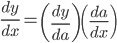:

The “chain rule can be extended” according to the complexity of the function. Here, if we substitute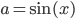.

How to do it?:
Broadly, there are two ways to do an automatic differentiation about which we will discuss later in detail :

• Forward Mode
• Backward Mode

There are “three important steps” to navigate through in order to do “Automatic Differentiation”:

• Drawing the “Computational Graph”
• Making the “Table” (Wengert’s list)
• Finding the “Derivative” :

1. Forward Mode
2. Backward Mode

##### For the sake of explanation, am taking the following function as an example :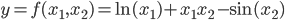with the values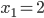and.

Step 1: Drawing the Computation Graph
1. Find out the Primitive Elements. The smallest possible element that makes sense in a function is called as the Primitive Element. In our case,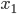and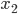are the primitive elements.
2. Give them some notation for simplicity of the graph. In our case,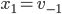and.
3. Now trace them to the next smallest possible element that makes sense. In our case, they are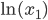,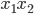and.
4. Give them some notation for simplicity of the graph. In our case,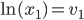,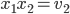and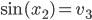. Butand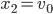. Therefore,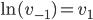,and. Therefore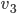,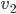,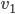, are the Parent elements of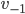,and they are the Child elements of,,.
5. Now trace them to the next smallest possible element that makes sense. In our case, it can either be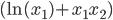and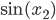orand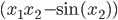. For the sake of the explanation, we are considering only the first possibility.
6. Give them some notation for simplicity of the graph. In our case, and as we know from previous steps,. But,,and. Therefore,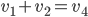. Therefore,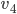is the Parent element of,and they are the child element of.
7. Now trace them to the next possible element that makes sense and in this case, it is the whole function.
8. Give them some notation for simplicity of the graph. In our case,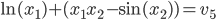. By substituting the appropriate terms from previous steps, we get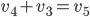.
9. Now draw a flowchart-like diagram that comprises all the previous steps. This should give us a graph like below and this is called a Computational Graph.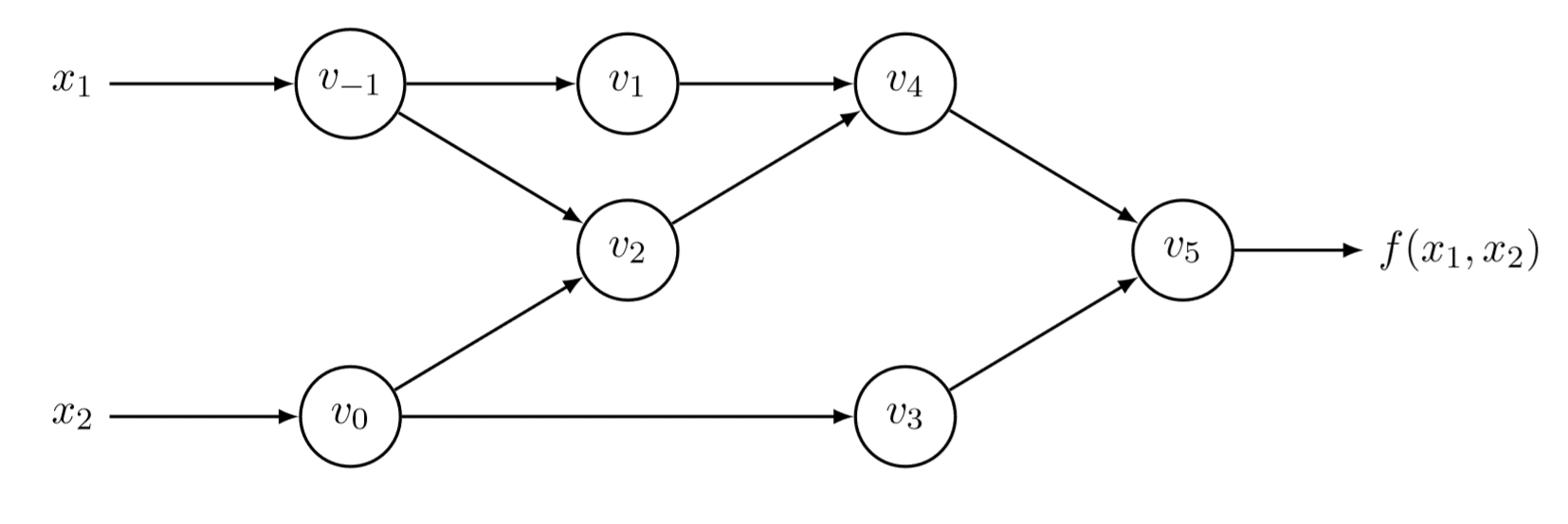Figure 1: Computational Graph; Credit: Baydin et al., 2015

These computational graphs are made on the go during the execution time and as a result, effectively increasing the efficiency of the process.

Step 2: Making the Table
1. Write down all the individual elements (Primal Trace) of the Computational Graph fromtowith their equivalents like following :Figure 2: Primal Trace Table; Credit: Baydin et al., 2015

Step 3: Finding the Derivative
This step is divided into two parts based on which mode we wish to proceed :

• Forward mode
• Backward mode

The two modes can be easily understood with respect to the chain rule that we saw before. Let’s consider the following chain rule,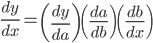In this, If we compute the first two elements (blue box) and then the third element (outer box) like showed in figure:3, then it is called as Forward mode.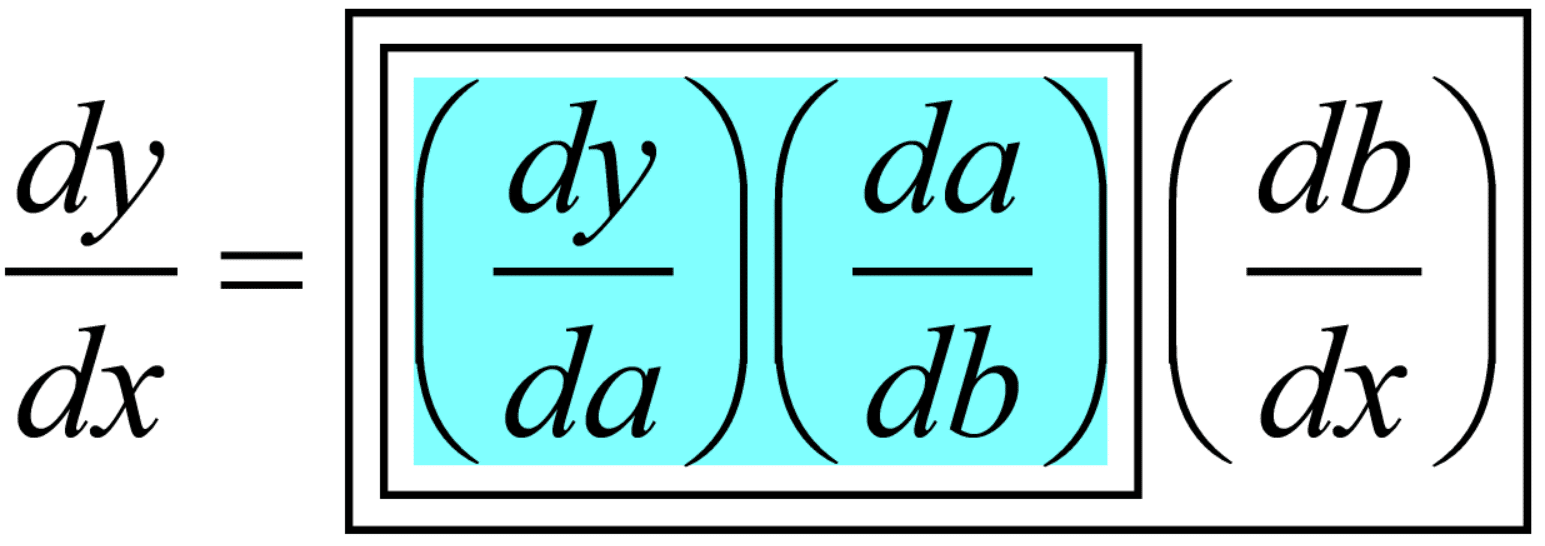Figure 3

If we compute the last two elements (blue box) and then the third element (outer box) like showed in figure:4, then it is called as Backward mode.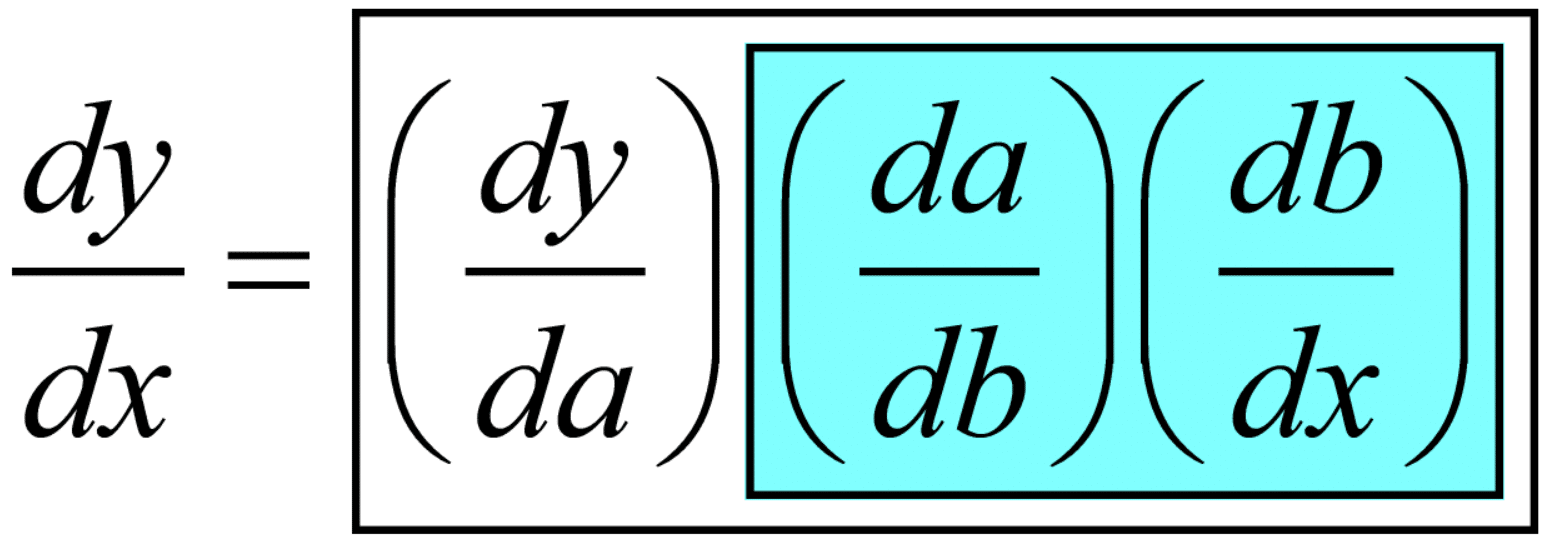Figure 4

Now let us find the derivates of the example function for both the modes.
Forward mode :
Find the derivatives of each element (Derivative trace) in the graph individually like shown in the following table. If you want to find the derivative with respect to, then set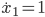and. If you want to find the derivative with respect tothen set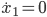and.Figure 5: Elements of the Computational graph [Left] and it’s respective derivatives (Derivative Trace) [Right] ; Credit: Baydin et al., 2015

##### Therefore, the value of derivative of the example function with respect toatandis 5.5 using Forward mode.

So, as we notice from the above procedure of Forward mode, by doing it one time, we were able to obtain the derivative with respect to. So, it becomes obvious that we need to repeat the process as many times the number of variables in the function which may still be efficient than the other methods but still is a redundant process and this is where the backward mode shines. Let’s dig deeper into it.

Backward mode :
Here making the Derivative trace is a bit more different compared to what we did in the forward mode. Take a look at the computational graph that we drew earlier for the example function. In the Backward mode, we are going to move from the final element of the computational graph toward the basic element. For example,andaccounts for the changes in. Similarly,andaccounts for the change inand SO ON. So, we are going to make a table (derivative trace) were the Child elements represents how much change they have brought into their Parent elements from Bottom to Top.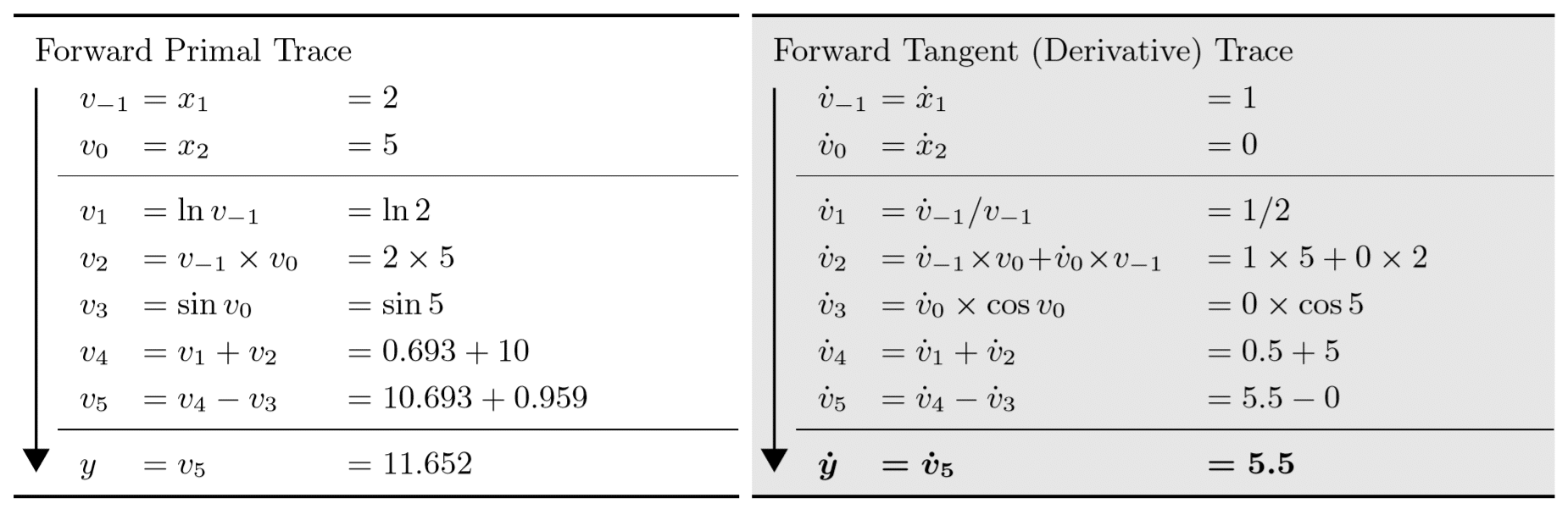Elements of the Computational graph [Left] and it’s respective derivatives (Derivative Trace) [Right] ; Credit: Baydin et al., 2015

##### Therefore the derivatives of the example function with respect toandatandare 5.5 and 1.716 respectively.

As you may have noticed, as compared to the repeat the process as many times the number of variables in the function situation in Forward mode, we got the derivatives with just one pass through the steps irrespective of the number of variables. This method of Backward mode automatic differentiation is exactly the same as back-propagation that we have been using in neural networks for so long, but this mathematical concept has been long hidden from the machine learning scientists until recently and the packages like Autograd which is the python implementation of it has rapidly increased the popularity of it in the machine learning community.

Popular Library: Autograd

##### As a result, the Backward mode of the Automatic Differentiation is more efficient than any other method that exists and is the ultimate solution for the problem of finding derivatives of functions of high complexity with ease in computers, acting as the best implementation for Neural Networks.

One more thing.
In a way, Numerical Differentiation can be considered as numerical in nature and Symbolic Differentiation can be considered as symbolic in nature. But Automatic Differentiation takes advantage of the best of both the worlds. Automatic Differentiation applies symbolic diﬀerentiation at the elementary operation level and numerical at the intermediate operation level.

References:
Research Papers :

• Baydin, A.G., Pearlmutter, B.A., Radul, A.A. and Siskind, J.M., 2017. Automatic differentiation in machine learning: a survey. Journal of machine learning research, 18(153), pp.1-153.
• Maclaurin, D., 2016. Modeling, inference and optimization with composable differentiable procedures (Doctoral dissertation).
• Maclaurin, D., Duvenaud, D. and Adams, R.P., 2015. Autograd: Effortless gradients in numpy. In ICML 2015 AutoML Workshop.

Videos :

• You Should Be Using Automatic Differentiation by Ryan Adams\
• The Simple Essence of Automatic Differentiation by Conal Elliott (A different approach in explaining Automatic Differentiation)
Advertisement